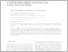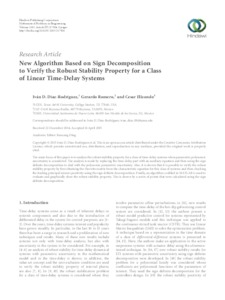# New Algorithm Based on Sign Decomposition to Verify the Robust Stability Property for a Class of Linear Time-Delay Systems

Díaz Rodríguez, Iván D. y Romero, Gerardo y Elizondo, Cesar (2015) New Algorithm Based on Sign Decomposition to Verify the Robust Stability Property for a Class of Linear Time-Delay Systems. Mathematical Problems in Engineering, 2015. pp. 1-8. ISSN 1024-123XVista previa
Texto

URL o página oficial: http://doi.org/10.1155/2015/217904

## Resumen

The main focus of this paper is to analyze the robust stability property for a class of time-delay systems when parametric polynomic uncertainty is considered. The analysis is made by replacing the time-delay part with an auxiliary equation and then using the sign definite decomposition to deal with the polynomic parametric uncertainty. Also, it is shown that it is possible to verify the robust stability property by first obtaining the Hurwitz matrix from the characteristic equation for this class of systems and then checking the leading principal minors positivity using the sign definite decomposition. Finally, an algorithm codified in MATLAB is used to evaluate and graphically show the robust stability property. This is shown by a series of points that were calculated using the sign definite de composition.

Tipo de elemento: Article
Divisiones: Ingeniería Mecánica y Eléctrica
Usuario depositante: Lic. Jesús E. AlvaradoVer elemento